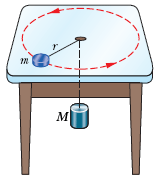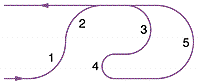# HELP! Physics PROBLEMSAnonymous

### Question Description

1.A puck of mass m = 1.14 kg slides in a circle of radius r = 20.0 cm on a frictionless table while attached to a hanging cylinder of mass M = 2.17 kg by a cord through a hole in the table. What speed keeps the cylinder at rest?

m/s2. In the figure below, a box of ant aunts (total mass m1 = 1.45 kg) and a box of ant uncles (total mass m2 = 3.45 kg) slide down an inclined plane while attached by a massless rod parallel to the plane. The angle of incline is θ = 30°. The coefficient of kinetic friction between the aunt box and the incline is μ1 = 0.226. The coefficient of kinetic friction between the uncle box and the incline is μ2 = 0.113.

(a) Compute the tension in the rod.
N

(b) Compute the common acceleration of the two masses.
m/s2

(c) How would the answers to (a) and (b) change if m2 trailed m1?3. The figure below shows an overhead view of an amusement park ride that travels at constant speed through five circular arcs of radii R0, 2R0, and 3R0. Rank the arcs according to the magnitude of the centripetal force acting on a rider while traveling in the arcs, greatest first. Use only ">" or "=" symbols. If any elements are equal, show their equality in numeric order (for example: 4>2=3)This question has not been answered.

Create a free account to get help with this and any other question!Brown University

1271 TutorsCalifornia Institute of Technology

2131 TutorsCarnegie Mellon University

982 TutorsColumbia University

1256 TutorsDartmouth University

2113 TutorsEmory University

2279 TutorsHarvard University

599 TutorsMassachusetts Institute of Technology

2319 TutorsNew York University

1645 TutorsNotre Dam University

1911 TutorsOklahoma University

2122 TutorsPennsylvania State University

932 TutorsPrinceton University

1211 TutorsStanford University

983 TutorsUniversity of California

1282 TutorsOxford University

123 TutorsYale University

2325 Tutors